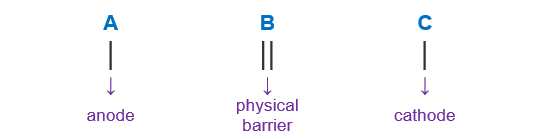# Problem: What is the shorthand notation that represents the following galvanic cell reaction?2 Fe2+(aq) + Cl2(g) → 2 Fe3+(aq) + 2 Cl-(aq)

###### FREE Expert Solution

We’re being asked to determine the cell notation for the redox reaction shown below:

2 Fe2+(aq) + Cl2(g)  → 2 Fe3+(aq) + 2 Cl-(aq)

When writing a cell notation, we use the following format – “as easy as ABCTo determine the cell notation of the reaction, we will use the following steps:

Step 1: Separate the reaction into two half-reactions.
Step 2: Determine the anode and the cathode.
Step 3: Write the cell notation of the reaction.

85% (425 ratings)###### Problem Details

What is the shorthand notation that represents the following galvanic cell reaction?

2 Fe2+(aq) + Cl2(g) → 2 Fe3+(aq) + 2 Cl-(aq)

Frequently Asked Questions

What scientific concept do you need to know in order to solve this problem?

Our tutors have indicated that to solve this problem you will need to apply the Cell Notation concept. If you need more Cell Notation practice, you can also practice Cell Notation practice problems.

What professor is this problem relevant for?

Based on our data, we think this problem is relevant for Professor Tomasik's class at CMU.